Computer Science Courses / Course / Chapter

## Test Prep Plan - Take a practice test

Take this practice test to check your existing knowledge of the course material. We'll review your answers and create a Test Prep Plan for you based on your results.
How Test Prep Plans work
1
Test your existing knowledge.
2View your test results
Based on your results, we'll create a customized Test Prep Plan just for you!
3Study smarter
Study more effectively: skip concepts you already know and focus on what you still need to learn.

# Graph Data Structures Chapter Exam

Exam Instructions:

Choose your answers to the questions and click 'Next' to see the next set of questions. You can skip questions if you would like and come back to them later with the "Go To First Skipped Question" button. When you have completed the practice exam, a green submit button will appear. Click it to see your results. Good luck!

Answered 0 of 30

### Page 1

#### Question 2 2. Suppose we are using Dijkstra's algorithm to find the shortest path from A to D in the graph shown. The first step is to mark the ending vertex, D, with a 0, label it as current and put a circle around it as shown. In the next step, we identify E and C as vertices that are connected by an edge to the current vertex. What would we mark vertex E and vertex C with, and what vertex would be the next current vertex in the algorithm?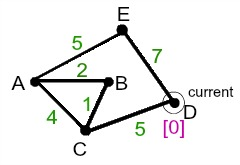### Page 2

#### Question 8 8. The following graph contains a negative edge. For this reason, the Dijkstra Algorithm will not compute the correct shortest path in one case. In which path would this error occur? (Let A be the source node)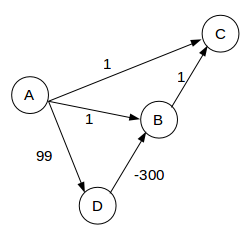#### Question 9 9. In the graph shown, the vertices represent cities, and the edges represent flights between those cities. The weights of the edges represent the cost of the flights, in hundreds of dollars. Based on this, what two cities are the cheapest to fly between, and what is the cost?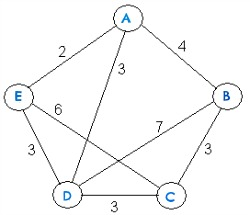#### Question 10 10. Suppose that a dating service is determining couples that would make a good match. In the graph shown, two sets of clients are represented with vertices, and the edges between the vertices indicate that the clients make a good match. Based on the graph, how many possible matches are there for Erin?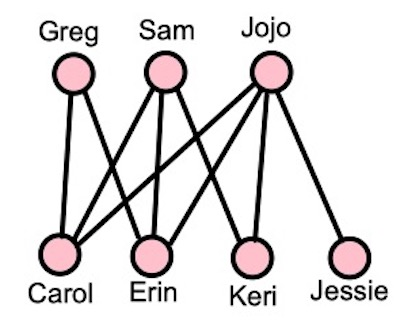### Page 3

#### Question 13 13. How many bridges does this graph have?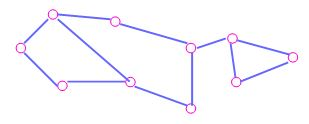#### Question 14 14. How many vertices does this graph have?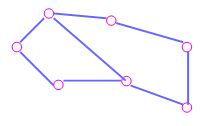#### Question 15 15. What is the shortest path length you can use to get from point 6 to point 2?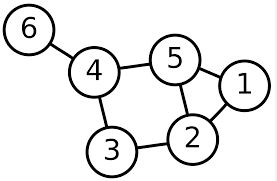### Page 4

#### Question 16 16. How many edges does this graph have?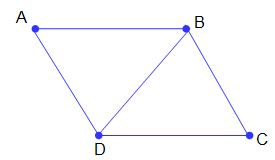#### Question 17 17. Which type of graph is this?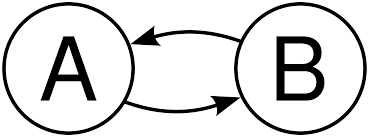#### Question 20 20. Which type of graph is the following?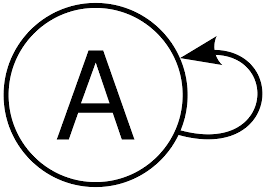### Page 5

#### Question 21 21. Which of the following will get you from point 3 to point 5?#### Question 23 23. How many vertices are in this graph?#### Question 24 24. How many edges does this graph have?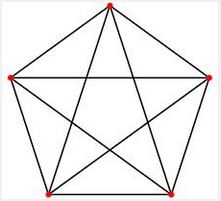#### Question 25 25. Suppose we just performed Dijkstra's algorithm on the graph shown to find the shortest path from vertex A to vertex C, so all of the vertices have been visited as indicated with the X ' s, including the starting vertex of A. Based on the graph and all of its final marks, what is the length of the shortest path from vertex A to vertex C?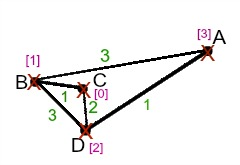### Page 6

#### Question 28 28. How many components does this graph have?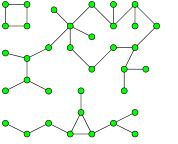#### Question 30 30. How many vertices are in this graph?#### Graph Data Structures Chapter Exam Instructions

Choose your answers to the questions and click 'Next' to see the next set of questions. You can skip questions if you would like and come back to them later with the "Go To First Skipped Question" button. When you have completed the practice exam, a green submit button will appear. Click it to see your results. Good luck!

Computer Science Courses / Course / Chapter

Support